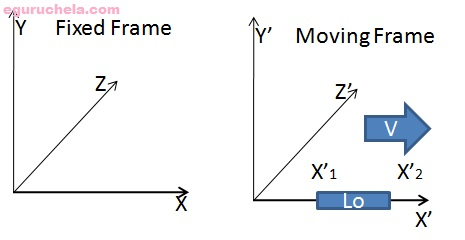# Length contraction

Length contraction is the phenomenon of a decrease in length of an object as measured by an observer who is traveling at any non-zero velocity relative to the object. This contraction is usually only noticeable at a substantial fraction of the speed of light.

$$L = Lo \sqrt{ 1 - \frac {v^2}{c^2}}$$

where

• Lo is the proper length (the length of the object in its rest frame),
• L is the length observed by an observer in relative motion with respect to the object,
• v is the relative velocity between the observer and the moving object,
• c is the speed of light,
• and the Lorentz factor, γ(v), is defined as

$$L = \frac {Lo}{\gamma}$$

In this equation it is assumed that the object is parallel with its line of movement.Go to index page